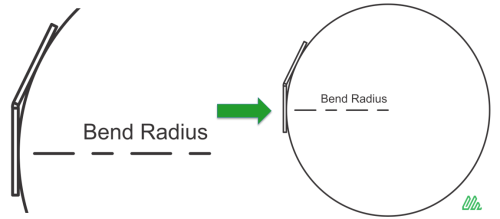The SAA Bend Angles diagnostic in SAARecorder provides a listing of the bend radii for each vertex (joint) in a ShapeArray. Bend radius is a measure of the radius of curvature and is a determining factor for how easy it will be to recover a ShapeArray from its conduit. Bend radii are indicators of how bent the conduit is and can be used to determine whether the rigid segments of the ShapeArray will be able to navigate the bends. More formally, the bend radius is defined as the radius of a circle that is drawn with two adjacent segments tangentially touching it as shown in the figure below.Figure 1. Bend radius is the radius of a circle that is drawn with two adjacent segments tangentially touching it